# Relativity and Black Holes General Relativity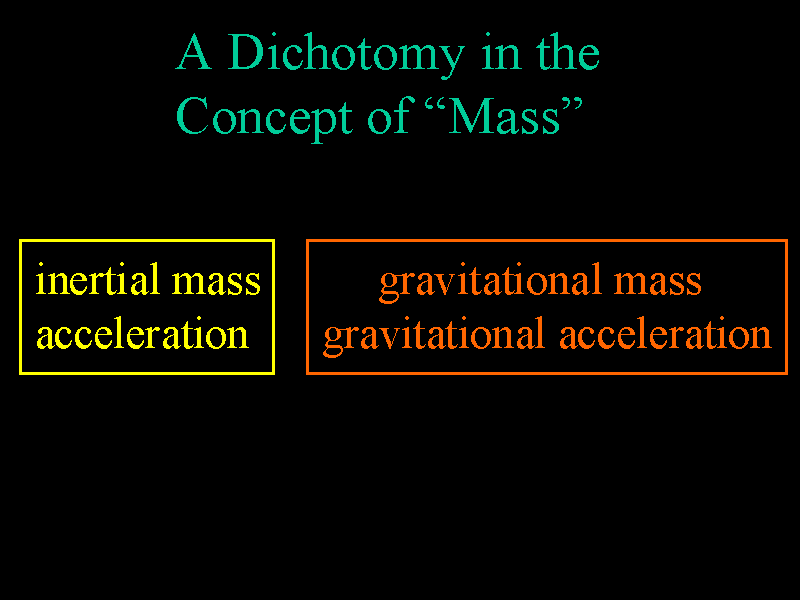Einstein was bothered by what he saw as a dichotomy in the concept of "mass." On one hand, by Newton's second law (F=ma), "mass" is treated as a measure of an objects resistance to changes in movement. This is called inertial mass. On the other hand, by Newton's Law of Universal Gravitation, an object's mass measures its response to gravitational attraction. This is called gravitational mass. As we will see, Einstein resolves this dichotomy by putting gravity and acceleration on an equal footing.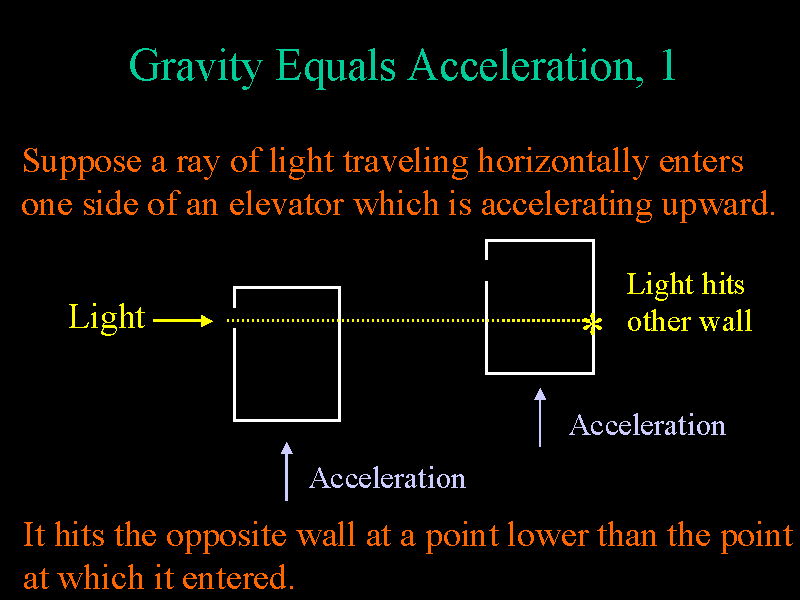Consider an observer in a windowless elevator which is accelerating upward. Suppose a ray of light enters near the top of the elevator on one side. Then while the light travels from one side of the elevator to the other, the elevator will accelerate upward and therefore the light will hit the opposite wall at a point lower than the point at which it entered.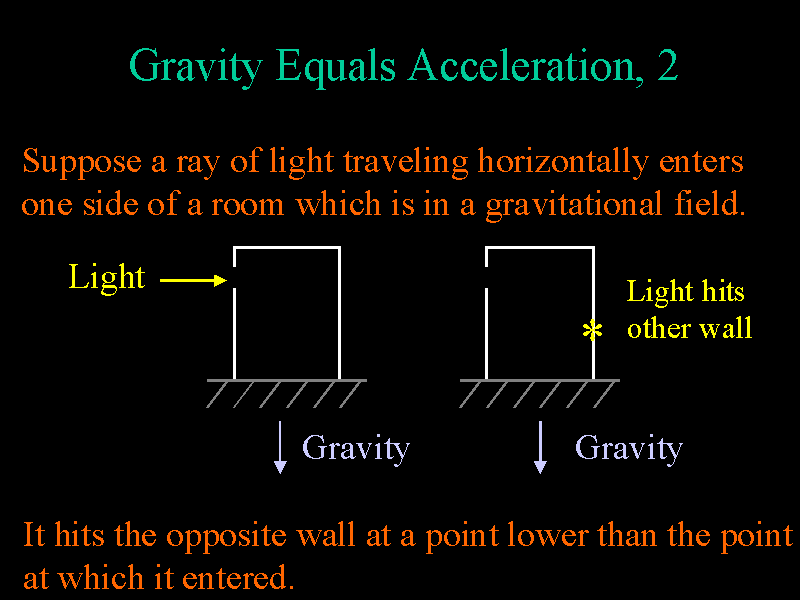Next, suppose the elevator is stationary, but in a gravitational field. Then if we put gravity and acceleration on an equal footing, we would expect the same outcome of our experiment involving light rays. That is, if a ray of light enters near the top of the stationary elevator on one side, we expect the light to hit the opposite wall at a point lower than the point at which it entered the elevator. In a sense, the light "falls" under the influence of gravity. Notice, then, that an occupant of the elevator could not tell if he were in a rapidly accelerating elevator, or if he were in a stationary elevator in a gravitational field.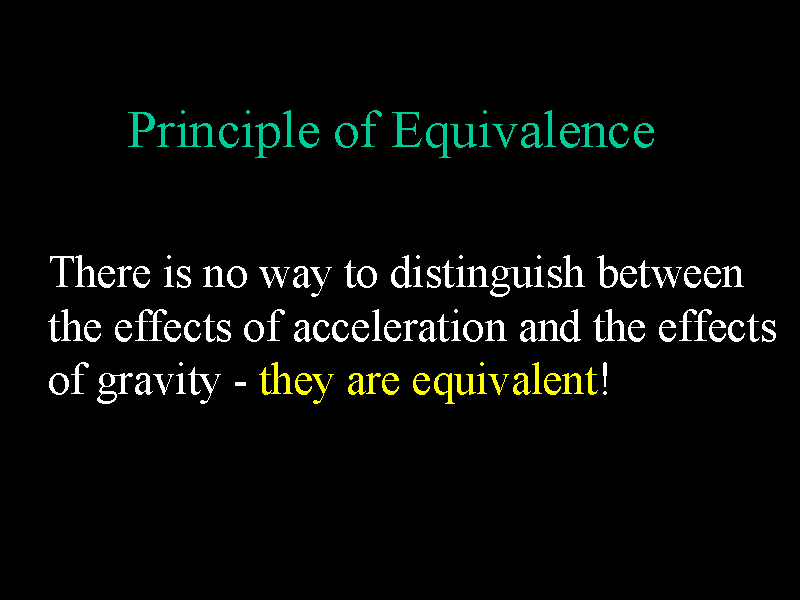Einstein summarizes this in his Principle of Equivalence: There is no way to distinguish between the effects of acceleration and the effects of gravity - they are equivalent!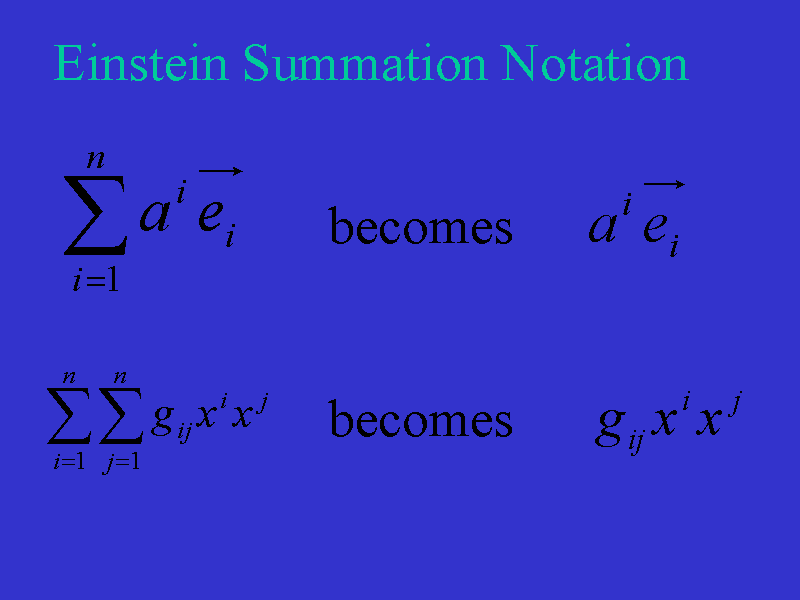In our technical exploration of general relativity, we will adopt Einstein's summation notation in which an index of summation appears in each term both as a subscript and as a superscript and the sigma summation symbol is omitted. Therefore, the sum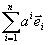becomes just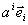. The double sum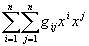becomes simply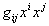. The range of the index of summation will be clear from the context (usually 0 to 3).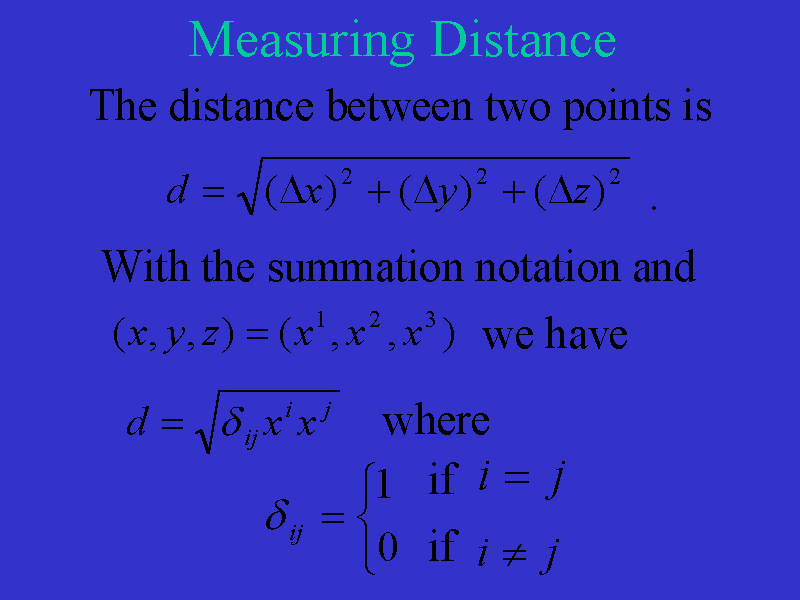In our standard 3-dimensional Euclidean space, we measure the distance between two points as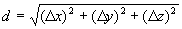If instead of using x, y, z to represent coordinates, we use x1, x2, x3, then with Einstein's summation notation, distance is calculated as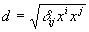where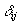is the Kronecker delta which takes on the value 1 if i and j are equal and the value 0 if i and j are different.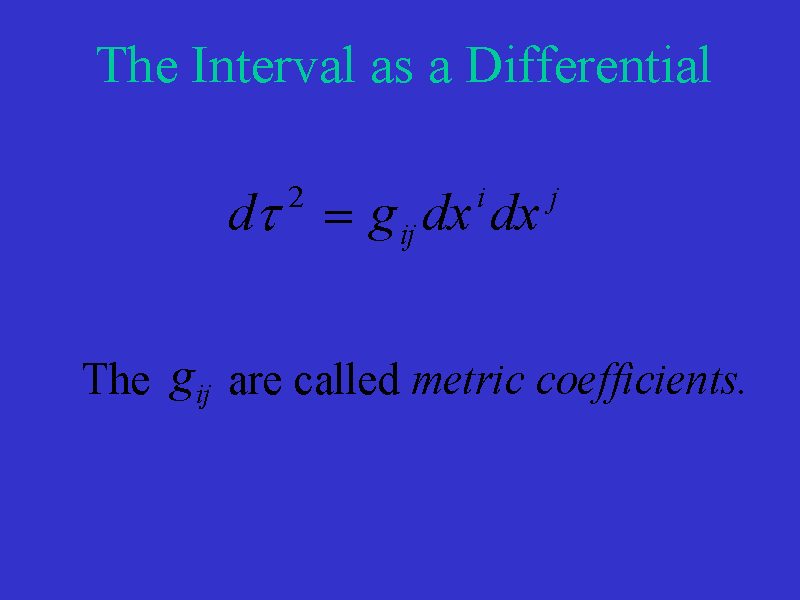Instead of simply measuring the distance between points in spacetime, we will be interested in differentials of the interval and the coordinates of spacetime. In a "curved" spacetime, we will measure the quantity as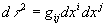. The curvature of the space will be determined by the gij (which are called metric coefficients) and the gij will be functions of space, time, and mass distribution.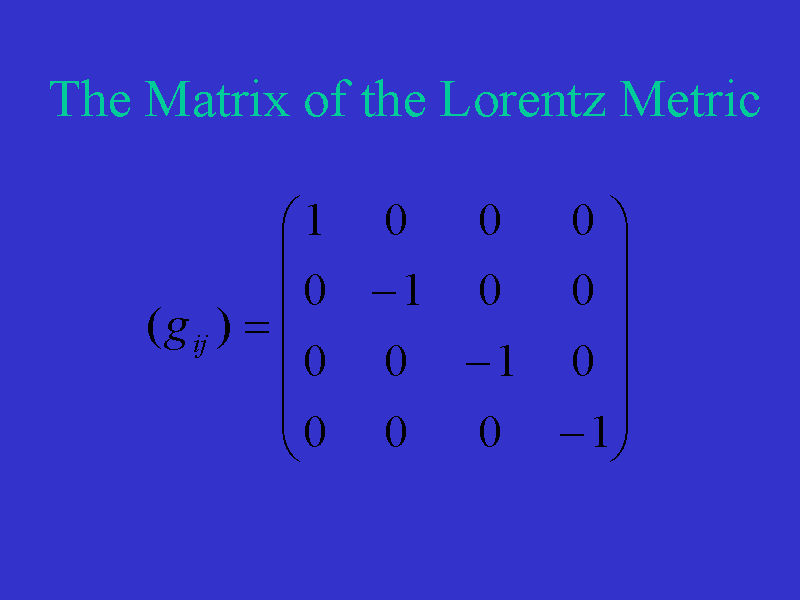As we have seen, in the absence of matter and with (t,x,y,z) = (x0,x1,x2,x3) the metric coefficients determine the matrix of the Lorentz metric seen here.

Now let's introduce some of the notation required to understand Einstein's field equations.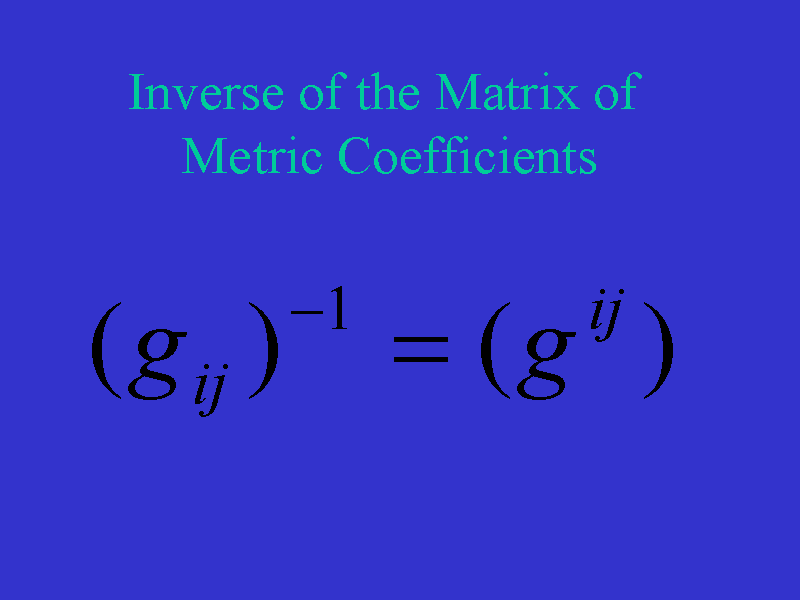First, we define the entries of the inverse of the matrix of metric coefficients as gij. The gij and gij and their partial derivatives are used to define the Christoffel symbols,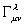.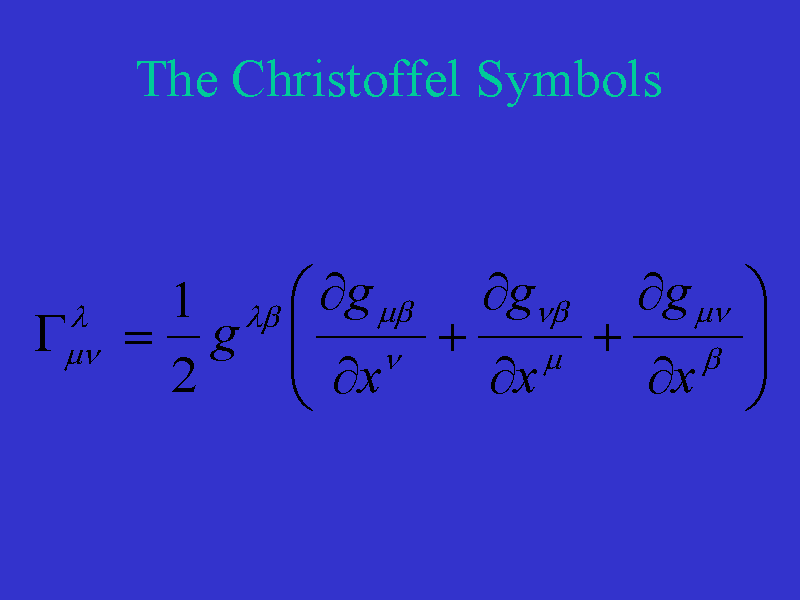Notice that these are functions of the gij's and partial derivatives of the gij's: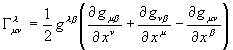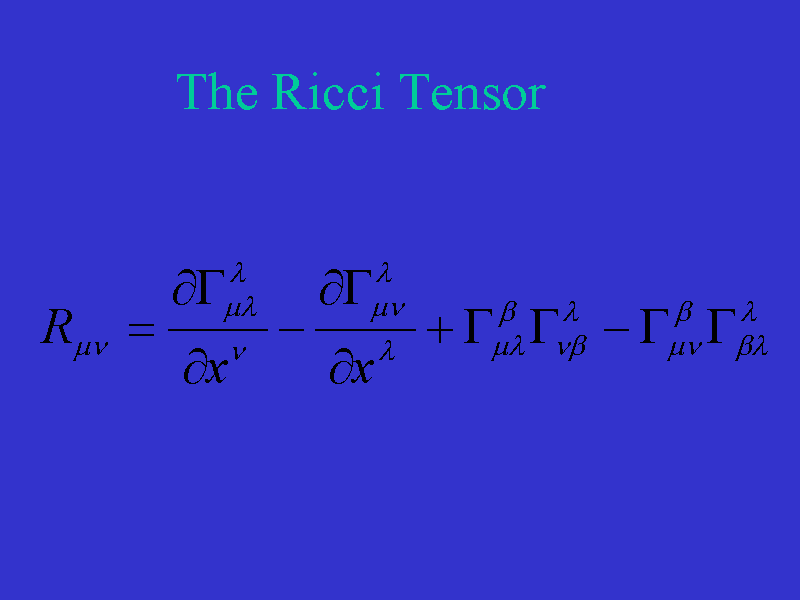The Ricci tensor is defined, in turn, in terms of the Christoffel symbols as seen here.

Einstein chose as his field equations of general relativity, the following: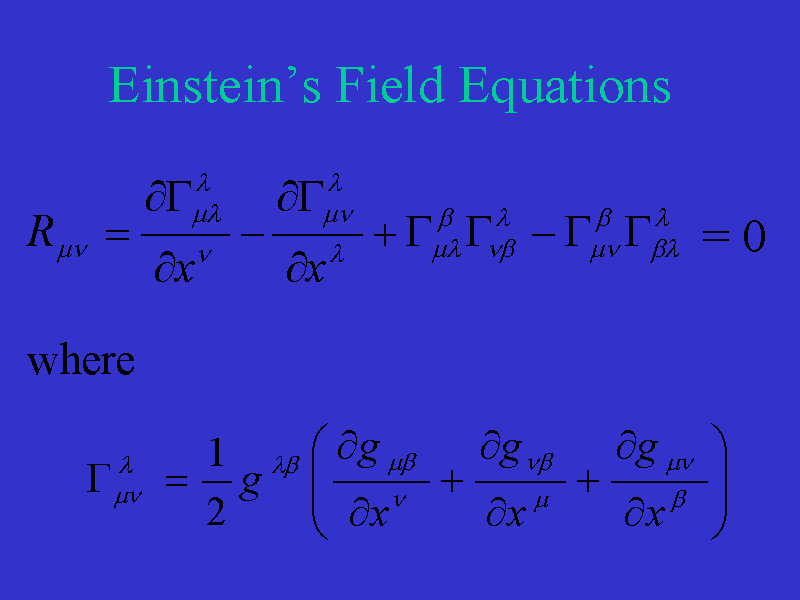That is, he chose for his field equations to set the Ricci tensor equal to zero. Since mu and nu range from 0 to 3, there are 16 different equations here.

We can simplify this notationally as: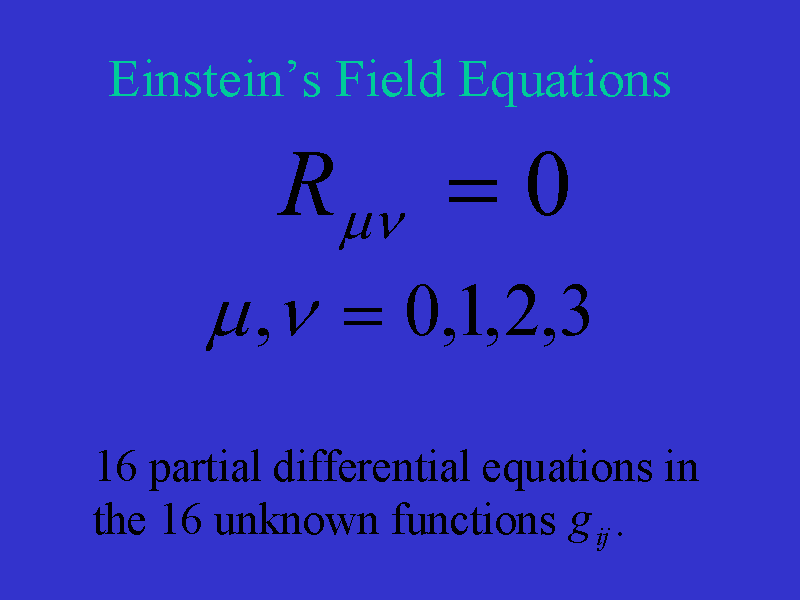So we see that Einstein's field equations are a system of 16 second order partial differential equations in the 16 unknown functions gij.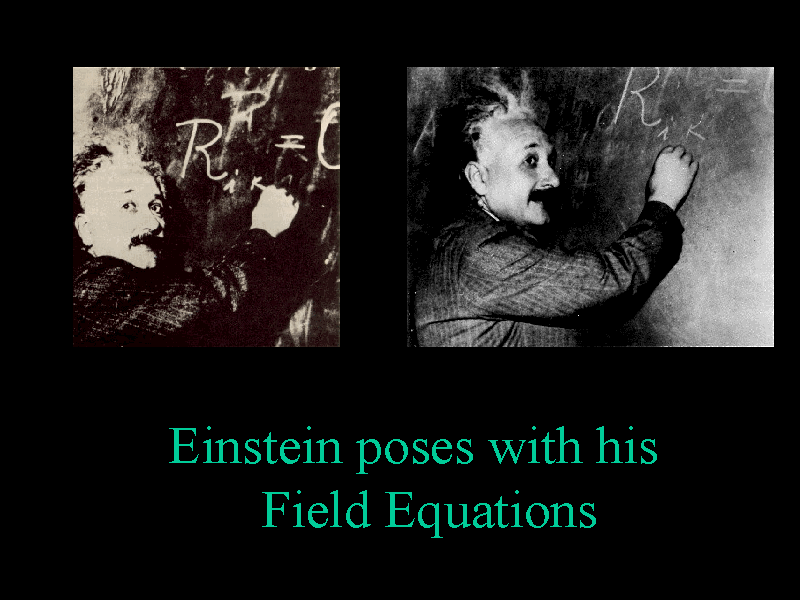Here we see Einstein posing with his field equation. The gij determine the metric form of spacetime and therefore all {\it intrinsic} properties of the 4-dimensional spacetime in which we live. In particular, curvature is determined by the Riemann-Christoffel curvature tensor.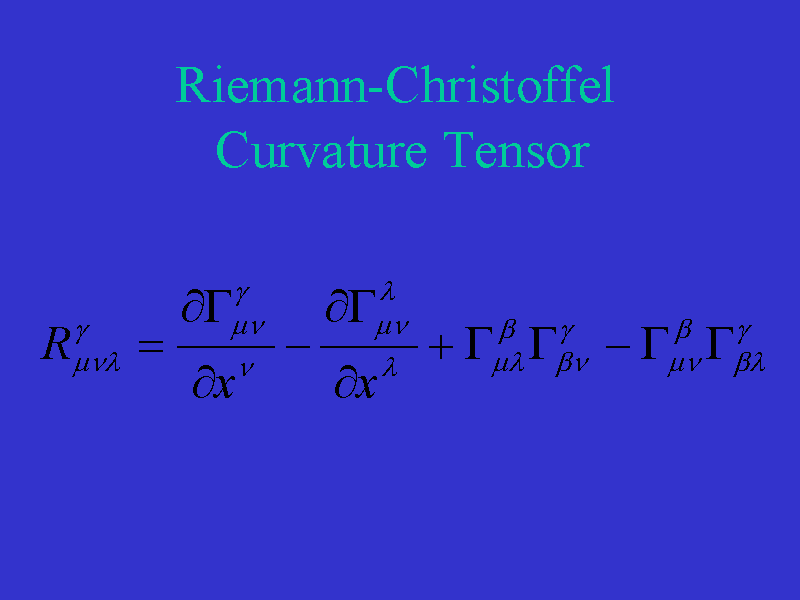Notice that mu, nu, lambda, and gamma range from 0 to 3 and therefore the curvature tensor consists of 256 entries, only 20 of which are independent.

Go to next section.

